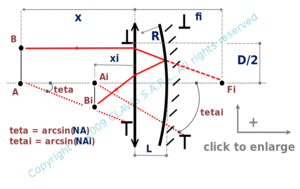and orgeometrical optics
paraxial conjugation

conjugation for a given object and a thin lens-spherical mirror combination

Caution
Fill in all input cells.
Choose all parameters unit.
Except L which is positive, distances and raddii are algebric.
Positive directions are according the arrows in the bottom right of the scheme.
As light rays pass through the lens twice ( before and after reflexion on the mirror ), fi sign can be confuzing. Sign is positive for converging lens and negative for diverging lens.

InputValueunit
R
fi
L
x
OutputValueUnit
xito be calculated
mto be calculatedR : radius of curvature of the mirror fi : effective focal length of the lens L : distance between the lens and the mirror vertex x : distance from the lens to the object plane xi : distance from the lens to the image plane

Note
This calculation page can not detect all unrealistic inputs.